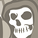# QlikView App Dev

Discussion Board for collaboration related to QlikView App Development.

Announcements
Live chat with experts, bring your API Integration questions. June 15th, 10 AM ET. REGISTER TODAY
cancel
Showing results for
Search instead for
Did you mean:Contributor II

## Complex aggr

Hi,

i need an advise:

My table :

DATETIME            ENERGY          DEVICE

14/8/12  00:00          1                         1

14/8/12  01:00          2                          1

14/8/12  02:00          3                         1

.

.

.

15/8/2012

.

.

.

.

16/8/2012  00:00          -1                         1

16/08/12  01: 00          26                         1

16/08/12  07: 00          35                         1

There are many DEVICE

DATETIME            ENERGY          DEVICE

14/8/12  00:00          1                         2

14/8/12  01:00          2                          2

14/8/12  02:00          3                         2

.

.

.

15/8/2012

.

.

.

.

16/8/2012  00:00          -1                         2

16/08/12  01: 00          99                         2

16/08/12  07: 00          110                         2

i need

the SUM of (max(Energy)) of today

Sutraction

the SUM of (Min(ENERGY) where Min Energy >0 )  of today

all the device sum together.

In this case: DEVICE 1 max of today is 35 and min of today(>0) is 26  ---> 35-26=6

DEVICE 2 max of today is 110 and min of today(>0) is 99 ----> 110-99 = 11

FINAL RESULT : 11+6 = 17

Is this possible ?

Thanks

9 RepliesCreator III

Can you split Datetime in 2 ? Date at time? Or create in script the Date with a floor(Datetime) ? Or (better) calculate a flag CURRENT_DATE (only if you refresh data everyday)

a graph with Device as dimension and expression:

max({\$<DATE={"\$(=Today())}"}>}ENERGY) - min({\$<DATE={"\$(=Today())}"}, ENERGY={">0"}>}ENERGY)

or

max({\$<CURRENT_DATE={"Yes"}>}ENERGY) - min({\$<CURRENT_DATE={"Yes"}, ENERGY={">0"}>}ENERGY)MVP

Hi

If I have understood you correctly, something like this expression should work (assuming there are no other dimensions not mentioned in your post)

Sum(Aggr(Max(ENERGY) - Min(If(ENERGY > 0, ENERGY, 0)), DATETIME, DEVICE))

Hope that helps

Jonathan

Logic will get you from a to b. Imagination will take you everywhere. - A EinsteinMVP

Try

=sum(

aggr(

max({<Date = {'\$(=today())'}>}ENERGY) - min({<ENERGY ={">0"},Date={'\$(=today())'}>}ENERGY)

,DEVICE)

)

where I created Date field like

LOAD

...

date(daystart(DATETIME)) as Date

...

FROM ...;

Hope this helps,

StefanContributor II
Author

Can i change the expression using Date > Daystart (today()) ? If i'll do an error appeared

So must not change the date with the daystart

=sum(

aggr(

max({<Date > {'\$(=today())'}>}ENERGY) - min({<ENERGY ={">0"},Date={'\$(=today())'}>}ENERGY)

,DEVICE)

)MVP

You need to use the equal sign here, it's more like a set assignment than a comparision operator.

But you can probably use

{<Date = {">\$(=today())'"}>}

i.e. an advanced search string (using double quotes) and a larger than operator in the search string.Contributor II
Author

i wrote

=sum(aggr( max({<DATETIME = {">\$(=today())'"}>}[ENERGY YELDED]) - min({<[ENERGY YELDED] ={">0"},DATETIME= {">\$(=today())'"}>}[ENERGY YELDED]),IDDEVICE))

but seems not work.

Is not possible just fix a value in dimension where i can make Qlik consider just the datetime > daystart(today())?MVP

I've noticed you are using the DATETIME field instead of the Date field. Field format is important in set analysis, so this could be a reason why it is not working.

You can use a calculated dimension to limit your Dates to dates in the future, but that will introduce another dimension, grouping your data by IDDEVICE and Date.

Is this what you want?Contributor II
Author

I have others field, DATETIME is diveded in YEAR,MONTH,DAY:

can i put in the expression YEAR = Year(Today()) , MONTH = Month(today()), DAY = Day(today()) ? or something like that ?

i'd like to avoid the creation of another dimension in the scriptMVP

You can format any date / time as you like using the QV date/time functions. If your DATETIME format is YYYYMMDD (please check the format codes in the Help), you can try something like

{<DATETIME = {">\$(=Date(today(),'YYYYMMDD'))'"}>}